Question

# Which of the following is a true statement for any population with mean μ and standard deviation σ? I. The distribution of sample means for sample size n will have a mean of μ.

Which of the following is a true statement for any population with mean μ and standard deviation σ? I. The distribution of sample means for sample size n will have a mean of μ. II. The distribution of sample means for sample size n will have a standard deviation of. III. The distribution of sample means will approach a normal distribution as n approaches infinity.

Which of the following is a true statement for any population with mean μ and standard deviation σ?

I.The distribution of sample means for sample size n will have a mean of μ.

True

II. The distribution of sample means for sample size n will have a

.standard deviation of σ/sqrt(n)    true

OR

standard deviation of σ   false

III. The distribution of sample means will approach a normal distribution as n approaches infinity.

True

Note:

The central limit theorem states that if we have a population with mean μ and standard deviation σ and take sufficiently large random samples from the population is taken , then the distribution of the sample means will be approximately normally distributed with mean μ and standard deviation σ/sqrt(n).

#### Earn Coins

Coins can be redeemed for fabulous gifts.

Similar Homework Help Questions
• ### A population of values has a normal distribution with μ = 134.3 and σ = 62.4 . You intend to draw a random sample of size n = 137 . What is the mean of the distribution of sample means? μ ¯ x = What is the standard deviation of the distrib

A population of values has a normal distribution with μ=134.3μ=134.3 and σ=62.4σ=62.4. You intend to draw a random sample of size n=137n=137.What is the mean of the distribution of sample means?μ¯x=μx¯= What is the standard deviation of the distribution of sample means?(Report answer accurate to 2 decimal places.)σ¯x=σx¯=

• ### 99 and standard deviation σ A population whose distribution is unknown has mean μ and a sample of...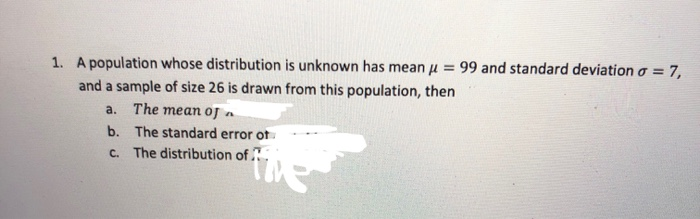99 and standard deviation σ A population whose distribution is unknown has mean μ and a sample of size 26 is drawn from this population, then 1, a. The mean oJ a b. The standard error ot c. The distribution of A population whose distribution is unknown has mean μ = 99 and standard deviation σ = 7 and a sample of size 26 is drawn from this population, then 1, a. b. c. The mean of X= The standard...

• ### A population has a mean μ=73 and a standard deviation σ=24. Find the mean and standard...

A population has a mean μ=73 and a standard deviation σ=24. Find the mean and standard deviation of a sampling distribution of sample means with sample size n=64.

• ### A population of values has a normal distribution with μ=89.8 and σ=85.9. You intend to draw a random sample of size n=13...

A population of values has a normal distribution with μ=89.8 and σ=85.9. You intend to draw a random sample of size n=131. What is the mean of the distribution of sample means? μx¯= What is the standard deviation of the distribution of sample means (i.e. the standard error)? (Report answer accurate to 2 decimal places.) σ¯x=

• ### As the sample size n increases, the shape of the distribution of the sample means taken...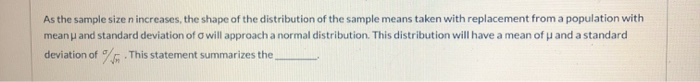As the sample size n increases, the shape of the distribution of the sample means taken with replacement from a population with mean and standard deviation of a will approach a normal distribution. This distribution will have a mean of u and a standard deviation of this statement summarizes the

• ### A random sample of size n = 64 is selected from a population with mean μ...

A random sample of size n = 64 is selected from a population with mean μ = 52 and standard deviation σ = 24. a. What will be the approximate shape of the sampling distribution of x? skewed symmetric normal b. What will be the mean and standard deviation of the sampling distribution of x? mean= standard deviation=

• ### drawn from a population with mean μ-52 and standard deviation σ= 4.3. Mou may find it...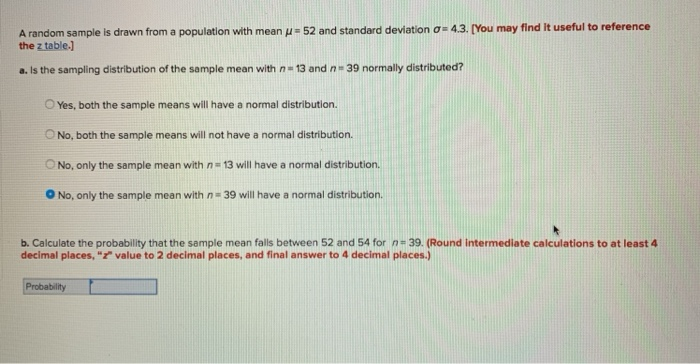drawn from a population with mean μ-52 and standard deviation σ= 4.3. Mou may find it useful to reference A random sample the z table.] a. Is the sampling distribution of the sample mean with n-13 and n# 39 normally distributed? ○Yes, both the sample means will have a normal distribution. O No, both the sample means will not have a normal distribution. O No, only the sample mean with n 13 will have a normal distribution. O No, only...

• ### 1 You draw a random sample ofsizen=16 from a population with mean μ 100 and standard...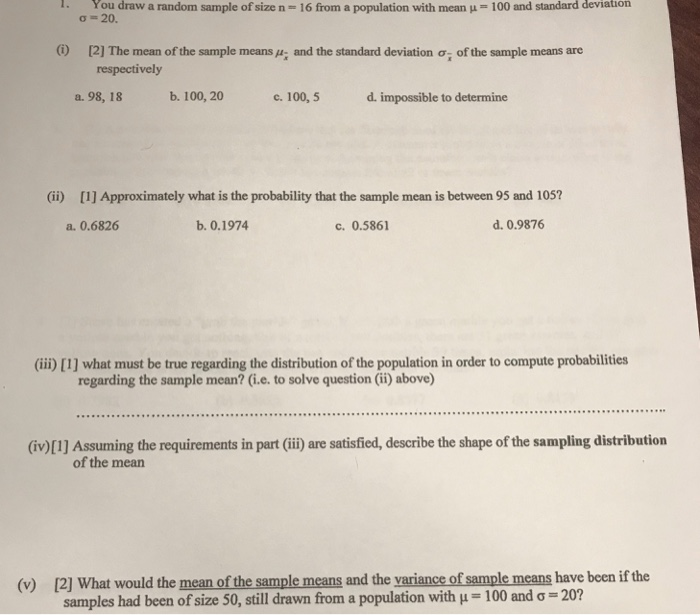1 You draw a random sample ofsizen=16 from a population with mean μ 100 and standard deviation ơ 20.  The mean of the sample means": and the standard deviation ơi of the sample means are respectively a. 98, 18 b. 100, 20 c. 100,5 d. impossible to determine (ii)  Approximately what is the probability that the sample mean is between 95 and 105? a. 0.6826 b. 0.1974 c. 0.5861 d. 0.9876 (ii)  what must be true regarding...

• ### A random sample of size 36 is to be selected from a population that has a mean μ = 50 and a standard deviation σ of 10

A random sample of size 36 is to be selected from a population that has a mean μ = 50 and a standard deviation σ of 10.    * a. This sample of 36 has a mean value of , which belongs to a sampling distribution. Find the shape of this sampling distribution.    * b. Find the mean of this sampling distribution.    * c. Find the standard error of this sampling distribution.    * d. What is the...

• ### What happens to the mean and standard deviation of the distribution of sample means as the size of the sample decreases?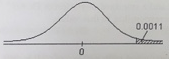47. What happens to the mean and standard deviation of the distribution of sample means as the size of the sample decreases? A) The mean of the sample means stays constant and the standard error decreases. B) The mean of the sample means increases and the standard error stays. C) The mean of the sample means decreases and the standard error increases. D) The mean of the sample means stays constant and the standard error increases. 48. Find the critical value ze that corresponds to...